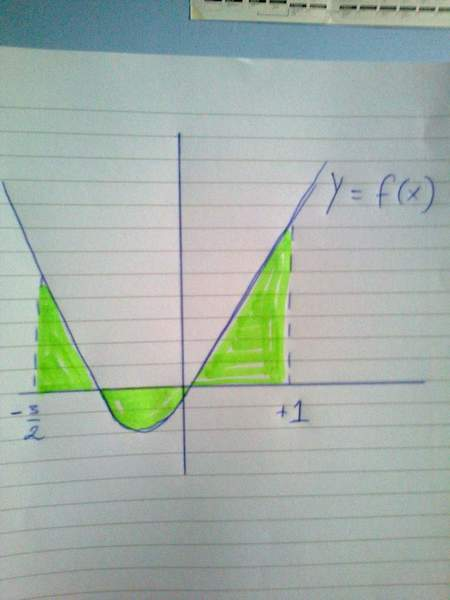# Integrating for area under a curve.

So, as you can see in the attached pictures, I needed to find the common area bound by the 2 curves.

Is the method I have outlined correct because according to the marking scheme it just says

$\int g(x)$ - $\int f(x)$ (The limits are the same, -3/2 to 1)

The way I see it, this does not account for the little bit below the x axis. Am I wrong because I always thought with these area questions you had to the 2 sides of an axis separately.

#### Attachments

Hi Darth Frodo. The marking scheme is correct because using that method will find the signed area between f(x) and g(x) regardless of whether it is above or below y=0. You can think of a normal integration as being ∫g(x)dx-∫0dx, this will find the area bound by 0 and g(x), obviously ∫0dx is just 0. For this case we replace 0 with f(x) because we want the area between f(x) and g(x) not 0 and g(x).

Ok so I found another problem with area above and below the axis,

Is this correct. The graph will trap the area between the limits regardless of the side of the axis its on right?

#### Attachments

So, as you can see in the attached pictures, I needed to find the common area bound by the 2 curves.

Is the method I have outlined correct because according to the marking scheme it just says

$\int g(x)$ - $\int f(x)$ (The limits are the same, -3/2 to 1)

The way I see it, this does not account for the little bit below the x axis. Am I wrong because I always thought with these area questions you had to the 2 sides of an axis separately.

for integral g(x) dx, dx is width of elementary strip and g(x) is its y coordinate or height above x axis. Integrating this will give you only area b/w g(x) and x axis.

Doing same for f(x)dx first integral, we can divide it into 3 parts, -1.5 to -1, -1 to 0, 0 to 1
The -1.5 to -1 and 0 to 1 parts wil remove extra area that was calculated by g(x) integral ( the area which we didnt need)
Now for -1 to 0 part, f(x) is negative, so integral will solve out to be negative. But you have a - outside too. So this wil result in positive area after all. Also, this is the area of f(x) and x axis below x axis.

Now third integral is also this is the area of f(x) and x axis below x axis.
But this time it will be negative, as there is + outside integration.
So this will remove the required area that was added by second integral. So by removing third integral, you will get correct answer.

But the second problem I did, with the single curve, was that correct? Because that was actually an example in the book and it said to split it up into 2 areas. But when I tried it with the limits 1 to 5 it was wrong...

I'm totally confused now.

Doing same for f(x)dx first integral, we can divide it into 3 parts, -1.5 to -1, -1 to 0, 0 to 1
The -1.5 to -1 and 0 to 1 parts wil remove extra area that was calculated by g(x) integral ( the area which we didnt need)
Now for -1 to 0 part, f(x) is negative, so integral will solve out to be negative. But you have a - outside too. So this wil result in positive area after all. Also, this is the area of f(x) and x axis below x axis.

So does that mean that ∫ f(x) between -1.5 and 1 captures the area shown in the pic below?

#### Attachments

SammyS
Staff Emeritus
Homework Helper
Gold Member
So does that mean that ∫ f(x) between -1.5 and 1 captures the area shown in the pic below?No. That integral will give the area of the green region that's above the x-axis minus the area of the green region that's below the x-axis.

Ok cool, thank you all for your help! This has been a little ill-defined in my head until now! Thanks to everyone!

It's always ∫(top curve)-(bottom curve) for this sort of problem. Just when the y=0 axis is the top/bottom, that integral disappears. This is what I meant by signed area. If you do ∫f(x)dx-∫g(x)dx where g is above f, then this will give you a negative area.

So does that mean that ∫ f(x) between -1.5 and 1 captures the area shown in the pic below?

Yes it will give the area above x axis as positive and below one as negative. This is because for limit -1 to 0, f(x) is negative

EDIT: Just like sammys said ...